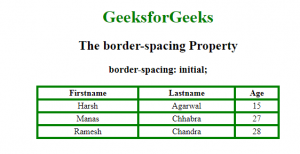# CSS border-spacing Property

The border-spacing Property is used to set the distance between the borders of neighboring cells in the Table. This property works only when the border-collapse property is set to no-collapse separate.

Default Value:

• 2px

Syntax:

`border-spacing: length|initial|inherit; `

Property values:

1. length-length: It is used to set the distance between the borders of adjacent cells. It does not allow negative values.
• If two values are defined, first value defines the horizontal space and second value defines the vertical spacing.
• If only one value is given then it defines the both horizontal and vertical spacing between the adjacent borders of cells.
2. initial: It sets the property to its default value.

Syntax:

`border-spacing:initial;`

Example:

## html

 `` `<``html``>`   `<``head``>` `    ``<``title``>border-spacing property` `    ``<``style``>` `        ``table,` `        ``th,` `        ``td {` `            ``border: 2px solid green;` `            ``text-align: center;` `        ``}` `        `  `        ``#geeks {` `            ``border-collapse: separate;` `            ``background-color: none;` `            ``border-spacing: initial;` `        ``}` `        `  `        ``h1 {` `            ``color: green;` `        ``}` `    ``` ``   `<``body``>` `    ``<``center``>` `        ``<``h1``>GeeksforGeeks` `        ``<``h2``> The border-spacing Property` `        ``<``h3``>border-spacing: initial;` `        ``<``table` `style``=``"width:70%"` `id``=``"geeks"``>` `            ``<``tr``>` `                ``<``th``>Firstname` `                ``<``th``>Lastname` `                ``<``th``>Age` `            ``` `            ``<``tr``>` `                ``<``td``>Harsh` `                ``<``td``>Agarwal` `                ``<``td``>15` `            ``` `            ``<``tr``>` `                ``<``td``>Manas` `                ``<``td``>Chhabra` `                ``<``td``>27` `            ``` `            ``<``tr``>` `                ``<``td``>Ramesh` `                ``<``td``>Chandra` `                ``<``td``>28` `            ``` `        ``` `    ``` ``   `                   `

Output:Supported Browsers: The browser supported by css border-spacing property are listed below: